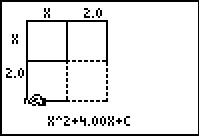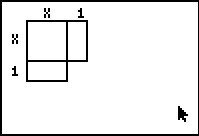# Activities

••• ##### Author9-12

40 Minutes

• ##### Device
• TI-83 Plus Family
• TI-84 Plus
• TI-84 Plus Silver Edition
•TI-84 Plus C Silver Edition

## Area of the Missing Square#### Activity Overview

Students explore the relationship between the value of b and c, in y = x2 + bx + c, form of the quadratic equation.

#### Key Steps

•Students will be introduced to the area model representation of a quadratic equation. The teacher will lead a discussion about the area of the missing square and its relationship to area of the whole square (x+1)2.

•In Problems 2 and 3, students will use this model to explore the relationship needed to complete the square of a quadratic trinomial. This exploration will occur with both integer and non-integer lengths. Students will write the Area equation for several different values of the displayed width.